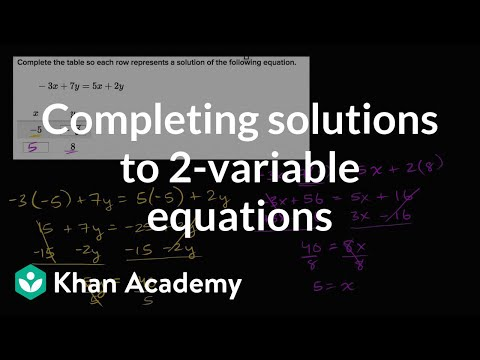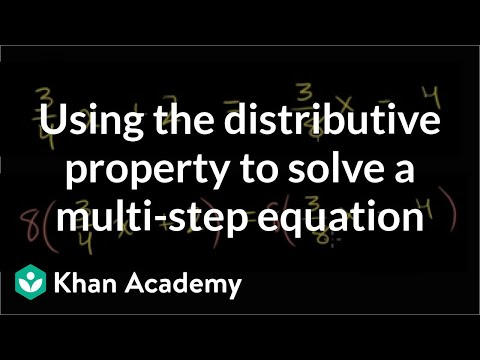Video

# Equations with parentheses (Full video)

Description: Sal solves the equation -9 - (9x - 6) = 3(4x + 6) using the distributive property. Created by Sal Khan and Monterey Institute for Technology and Education. We have the equation negative 9 minus this whole expression, 9x minus 6 is equal to 3 times this whole expression, 4x plus 6. So the left-hand side of our equation, we have our negative 9. And then we want to multiply the negative 1 times each of these terms.

### Other videos you might be interested in### Completing solutions to 2-variable equations (Full video)